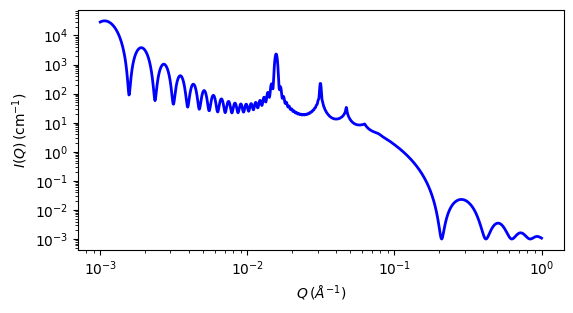# lamellar_stack_caille

Random lamellar sheet with Caille structure factor

Parameter

Description

Units

Default value

scale

Scale factor or Volume fraction

None

1

background

Source background

cm-1

0.001

thickness

sheet thickness

30

Nlayers

Number of layers

None

20

d_spacing

lamellar d-spacing of Caille S(Q)

400

Caille_parameter

Caille parameter

-2

0.1

sld

layer scattering length density

10-6-2

6.3

sld_solvent

Solvent scattering length density

10-6-2

1

The returned value is scaled to units of cm-1 sr-1, absolute scale.

This model provides the scattering intensity, $$I(q) = P(q) S(q)$$, for a lamellar phase where a random distribution in solution are assumed. Here a Caille $$S(q)$$ is used for the lamellar stacks.

Definition

The scattering intensity $$I(q)$$ is

$I(q) = 2\pi \frac{P(q)S(q)}{q^2\delta }$

The form factor is

$P(q) = \frac{2\Delta\rho^2}{q^2}\left(1-\cos q\delta \right)$

and the structure factor is

$S(q) = 1 + 2 \sum_1^{N-1}\left(1-\frac{n}{N}\right) \cos(qdn)\exp\left(-\frac{2q^2d^2\alpha(n)}{2}\right)$

where

\begin{align*} \alpha(n) &= \frac{\eta_{cp}}{4\pi^2} \left(\ln(\pi n)+\gamma_E\right) && \\ \gamma_E &= 0.5772156649 && \text{Euler's constant} \\ \eta_{cp} &= \frac{q_o^2k_B T}{8\pi\sqrt{K\overline{B}}} && \text{Caille constant} \end{align*}

Here $$d$$ = (repeat) d_spacing, $$\delta$$ = bilayer thickness, the contrast $$\Delta\rho$$ = SLD(headgroup) - SLD(solvent), $$K$$ = smectic bending elasticity, $$B$$ = compression modulus, and $$N$$ = number of lamellar plates (n_plates).

NB: When the Caille parameter is greater than approximately 0.8 to 1.0, the assumptions of the model are incorrect. And due to a complication of the model function, users are responsible for making sure that all the assumptions are handled accurately (see the original reference below for more details).

Non-integer numbers of stacks are calculated as a linear combination of results for the next lower and higher values.

The 2D scattering intensity is calculated in the same way as 1D, where the $$q$$ vector is defined as

$q = \sqrt{q_x^2 + q_y^2}$Fig. 49 1D plot corresponding to the default parameters of the model.

Source

References

1. F Nallet, R Laversanne, and D Roux, J. Phys. II France, 3, (1993) 487-502

2. J Berghausen, J Zipfel, P Lindner, W Richtering, J. Phys. Chem. B, 105, (2001) 11081-11088

Authorship and Verification

• Author: